# Time and quality stats for datasets of random cities

##### Eric P. Hanson

We will use TravelingSalesmanExact to compute the exact cost and compare to the estimated best costs found by TravelingSalesmanHeuristics with various settings of quality. First we load the packages and set up some plotting:

using TravelingSalesmanExact, MosekTools, GLPK, Gurobi
const MosekF = with_optimizer(Mosek.Optimizer, QUIET = true)
const GLPKF = with_optimizer(GLPK.Optimizer)
const env = Gurobi.Env()

Academic license - for non-commercial use only

const GurobiF = with_optimizer(Gurobi.Optimizer, env, OutputFlag = 0)
using TravelingSalesmanHeuristics
using TravelingSalesmanExact: euclidean_distance
using TravelingSalesmanBenchmarks
using Plots
using DataFrames
using Printf, Random, Statistics
Statistics.quantile(p::Number) = Base.Fix2(quantile, p)
gr(fmt=:svg)

function get_plt_coords(cities)
n = length(cities)
inc(a) = a == n ? one(a) : a + 1
return [cities[inc(j)] for j = 0:n], [cities[inc(j)] for j = 0:n]
end
TravelingSalesmanExact.plot_cities(cities; kwargs...) = plot(get_plt_coords(cities)...; kwargs...)
plot_cities!(cities; kwargs...) = plot!(get_plt_coords(cities)...; kwargs...)

function plot_tours(cities, pairs; kwargs...)
plts = []
colors = sequential_palette(0, length(pairs)+1)[2:end]
for (index, (tour, label)) in enumerate(pairs)
plt = plot_cities(cities[tour], label = label, linewidth = 2,
color = colors[index])
plot_cities!(cities; title="Comparison of tours", st=:scatter, label="City locations", markersize = 5, kwargs...)
push!(plts, plt)
end
return plts
end

plot_tours (generic function with 1 method)


## Datasets with 50 cities

We generate a cost matrix by choosing cities at random, although with a fixed seed for reproducibility:

Random.seed!(639);
N = 50
cities = [100*rand(2) for _ = 1:N]

50-element Array{Array{Float64,1},1}:
[11.7554, 11.5978]
[46.8999, 76.8242]
[86.7694, 87.7545]
[96.7649, 91.7422]
[40.9502, 11.4839]
[88.2692, 6.2183]
[70.4396, 89.062]
[92.873, 82.3338]
[68.3331, 34.377]
[20.5518, 34.6197]
⋮
[32.3448, 12.3754]
[53.5813, 33.1869]
[64.1045, 33.2997]
[19.213, 70.3944]
[62.1499, 23.4867]
[48.5462, 80.8571]
[39.4135, 62.9089]
[59.2164, 45.378]
[9.15706, 90.7963]


Now we will compute an optimal tour and cost, and compare to the cost of tours found by the tsp_solve function of TravelingSalesmanHeuristics at various choices of quality_factor.

function cost_quality_plots(cities; n = 15, qs = range(0, stop = 100, step = 5))
cost = [ euclidean_distance(cities[i], cities[j]) for i=1:N, j=1:N ]

(tour_glpk, cost_glpk), time_glpk, _ = @timed get_optimal_tour(cost, GLPKF)
(tour_mosek, cost_mosek), time_mosek, _ = @timed get_optimal_tour(cost, MosekF)
(tour_gurobi, cost_gurobi), time_gurobi, _ = @timed get_optimal_tour(cost, GurobiF)
@assert cost_glpk ≈ cost_mosek ≈ cost_gurobi
t_exact, c_exact = tour_glpk, cost_glpk
tour_plt = plot_tours(cities, [(t_exact, "Optimal tour ($(length(cities)) cities)")], title = "Optimal tour")[] df = DataFrame( begin (tour, c), time, _ = @timed solve_tsp(cost; quality_factor = q) if c < c_exact && !(c ≈ c_exact) @warn "c < c_exact" c c_exact end (quality = q, cost = c, time = time ) end for _ = 1:n for q in qs) cost_df = by(df, :quality, :cost => median, :cost => quantile(.25), :cost => quantile(.75) ) cost_plt = hline([c_exact], label="Exact cost") plot!(cost_df, cost_df, ribbon = (cost_df .- cost_df, cost_df .- cost_df), label = "Median cost over$n heuristic runs per quality_factor", xlabel="quality_factor", ylabel="Cost", title="Cost vs quality" )

time_df = by(df, :quality, :time => median, :time => quantile(.25), :time => quantile(.75) )
time_plt = hline([time_glpk], label="Time for exact computation with GLPK")
hline!([time_mosek], label="Time for exact computation with Mosek")
hline!([time_gurobi], label="Time for exact computation with Gurobi")
plot!(time_df, time_df,  ribbon = (time_df .- time_df, time_df .- time_df),
label = "Median time over \$n heuristic runs per quality_factor", xlabel="quality_factor", ylabel="Time", title="Time vs quality" )

end

foreach(display, cost_quality_plots(cities))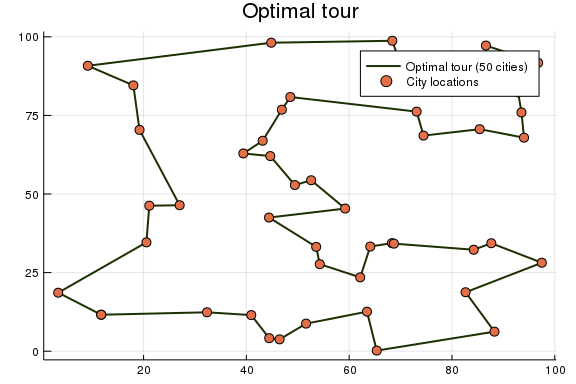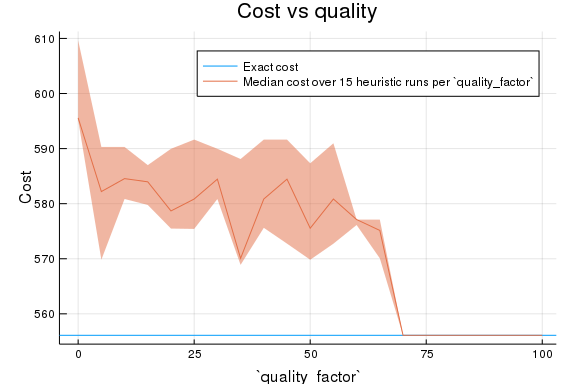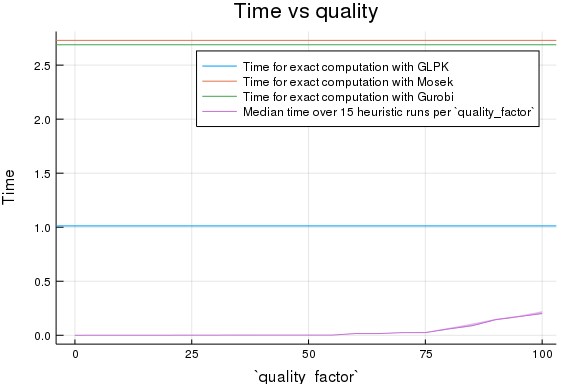Here, we see a visual diagram of the optimal tour, and a comparison of (tour) cost versus the quality_factor setting, and a similar comparison for the computation time. The ribbon shows the range between the first and third quartiles.

Let us repeat this for several different datasets each of 50 cities.

### Dataset 2

N = 50
cities = [100*rand(2) for _ = 1:N]
foreach(display, cost_quality_plots(cities))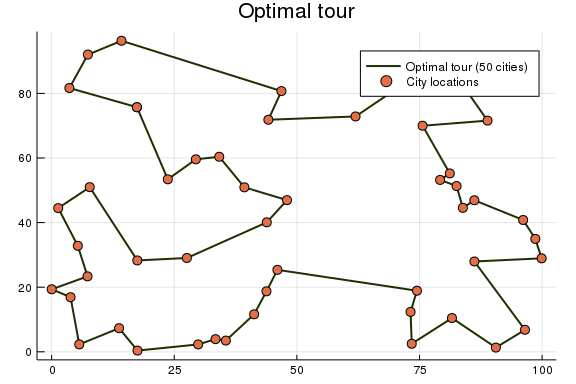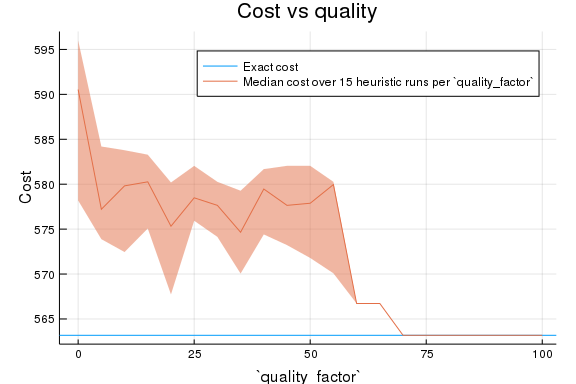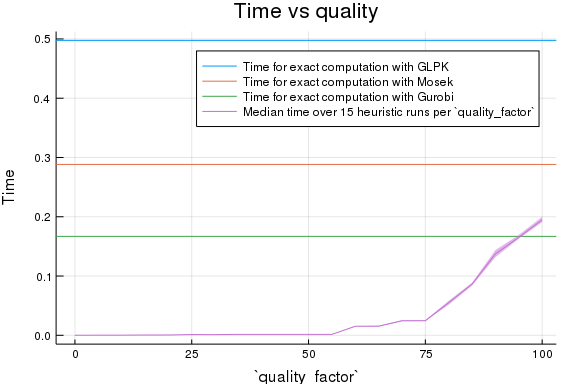### Dataset 3

cities = [100*rand(2) for _ = 1:N]
foreach(display, cost_quality_plots(cities))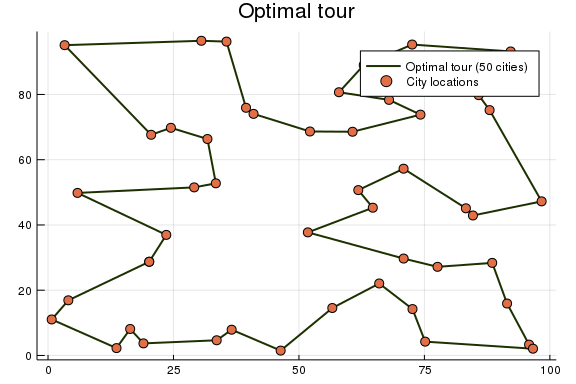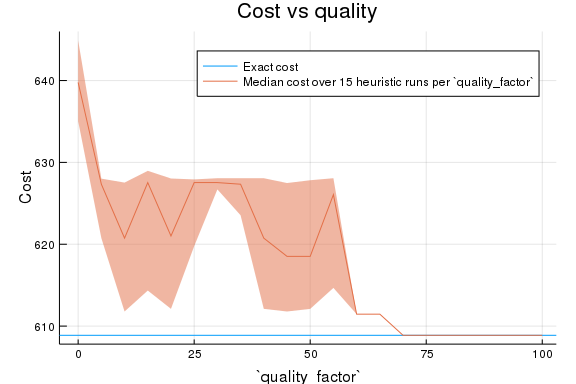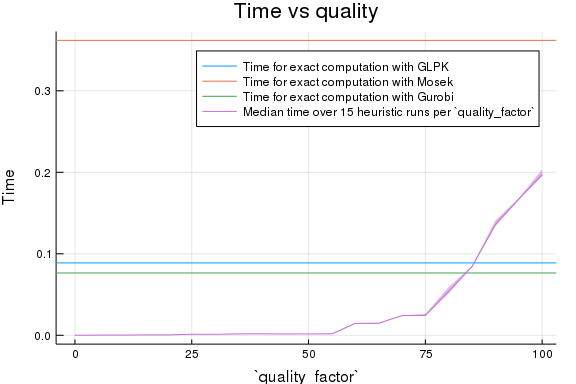### Dataset 4

cities = [100*rand(2) for _ = 1:N]
foreach(display, cost_quality_plots(cities))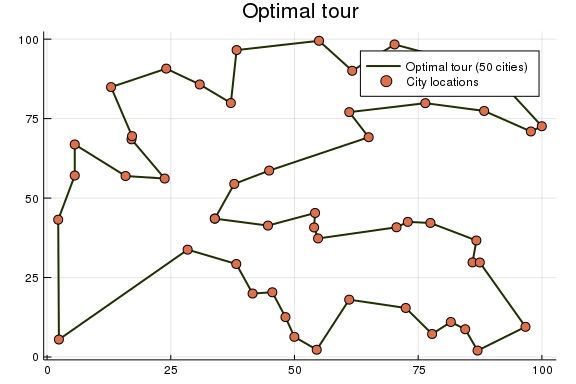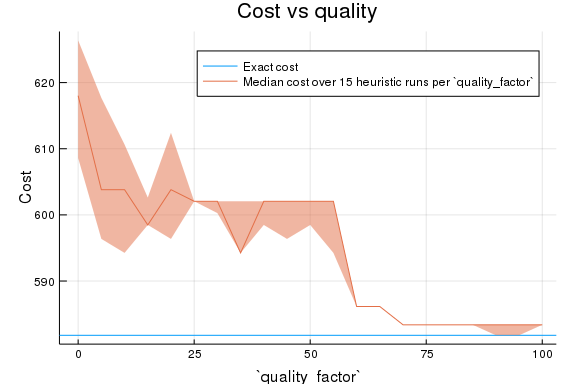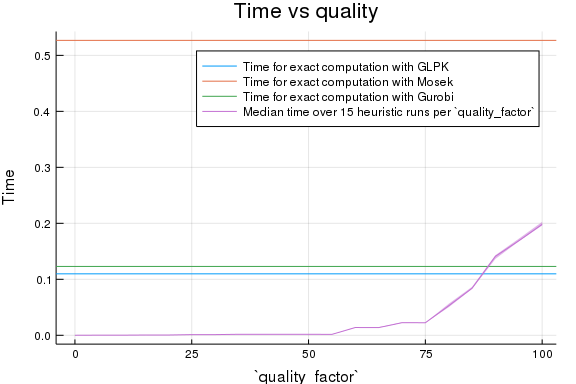### Dataset 5

cities = [100*rand(2) for _ = 1:N]
foreach(display, cost_quality_plots(cities))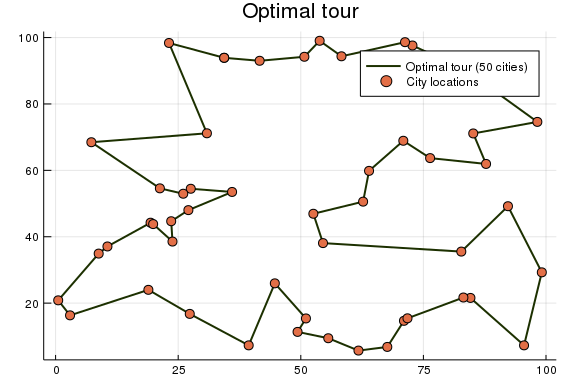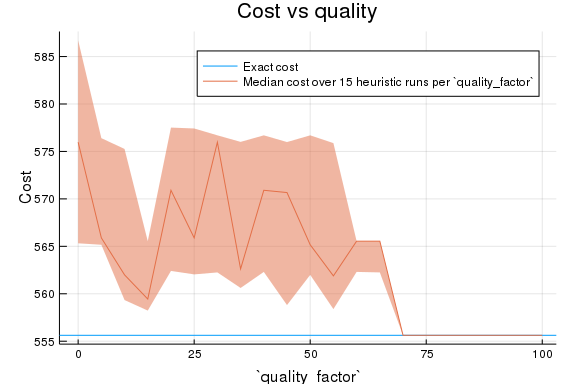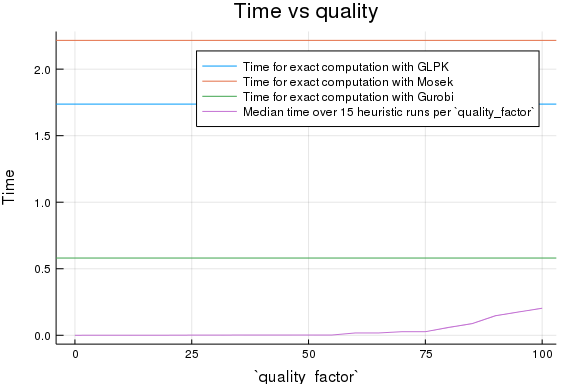## Datasets with more cities

We can try increasing the number of cities, although the runtime becomes very long.

### N = 80

N = 80
cities = [100*rand(2) for _ = 1:N]
foreach(display, cost_quality_plots(cities))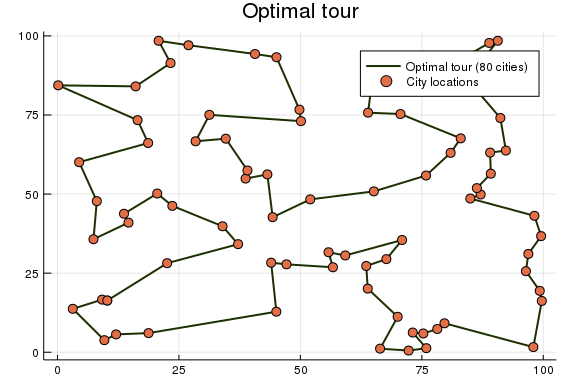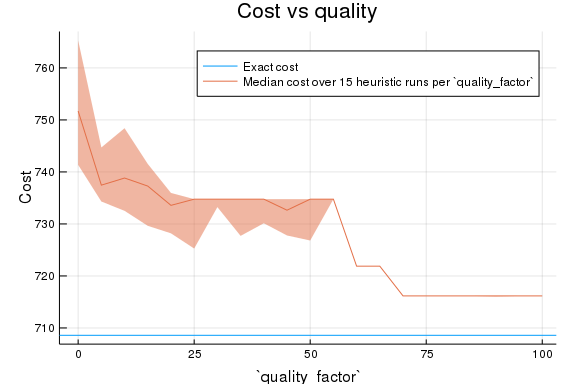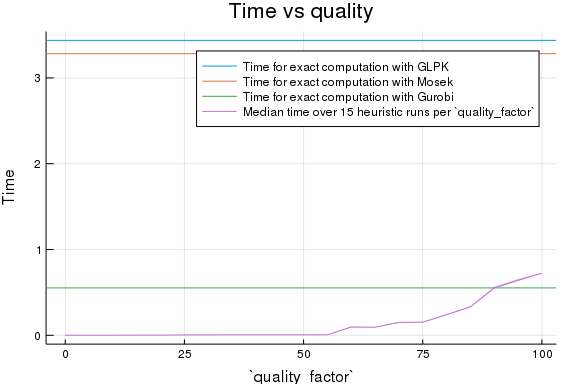## Appendix

These benchmarks are a part of the TravelingSalesmanBenchmarks.jl repository, found at: https://github.com/ericphanson/TravelingSalesmanBenchmarks.jl, based on the weave branch of DiffEqBenchmarks.

To locally run this tutorial, do the following commands:

using TravelingSalesmanBenchmarks
TravelingSalesmanBenchmarks.weave_file("random_50_cities_stats.jmd")

Computer Information:

Julia Version 1.1.0
Commit 80516ca202 (2019-01-21 21:24 UTC)
Platform Info:
OS: macOS (x86_64-apple-darwin14.5.0)
CPU: Intel(R) Core(TM) i5-5257U CPU @ 2.70GHz
WORD_SIZE: 64
LIBM: libopenlibm
Environment:
JULIA_EDITOR = "/Applications/Visual Studio Code.app/Contents/Frameworks/Code Helper.app/Contents/MacOS/Code Helper"


Package Information:

Status: /Users/eh540/Dropbox (Personal)/LinkedFolders/Julia/dev/TravelingSalesmanBenchmarks/Project.toml
[a93c6f00-e57d-5684-b7b6-d8193f3e46c0] DataFrames 0.17.1
[60bf3e95-4087-53dc-ae20-288a0d20c6a6] GLPK 0.9.1
[2e9cd046-0924-5485-92f1-d5272153d98b] Gurobi 0.6.0
[10745b16-79ce-11e8-11f9-7d13ad32a3b2] Statistics